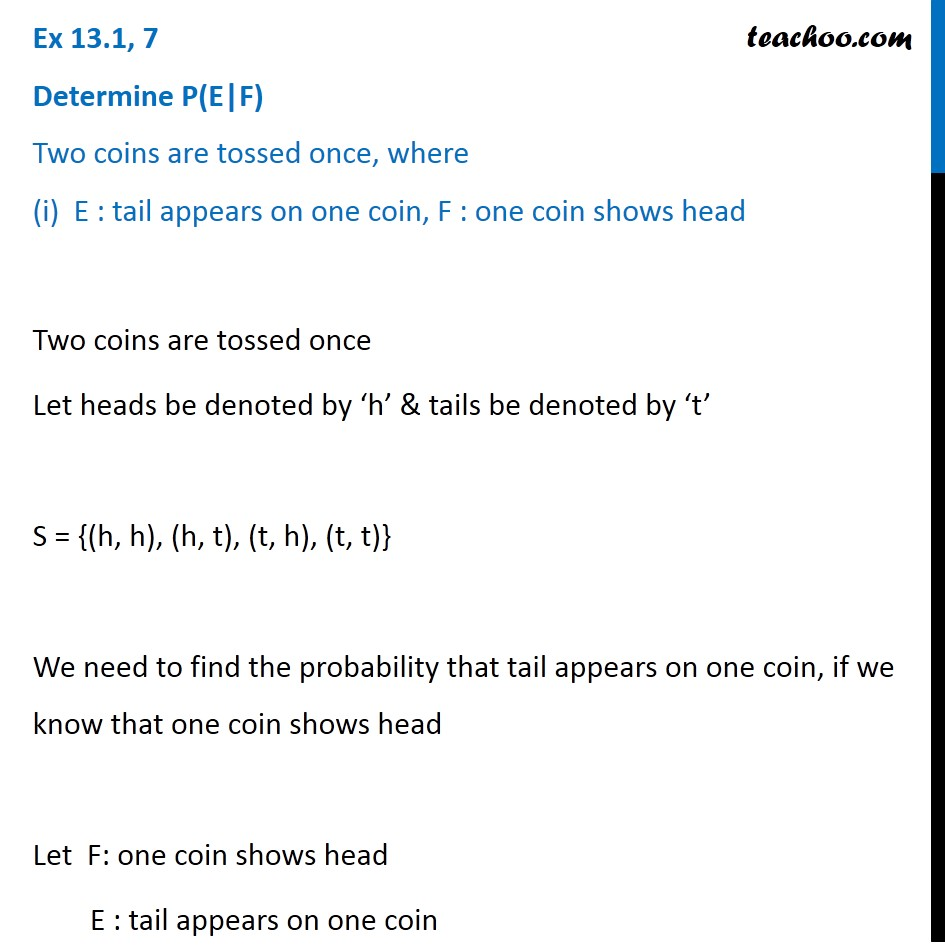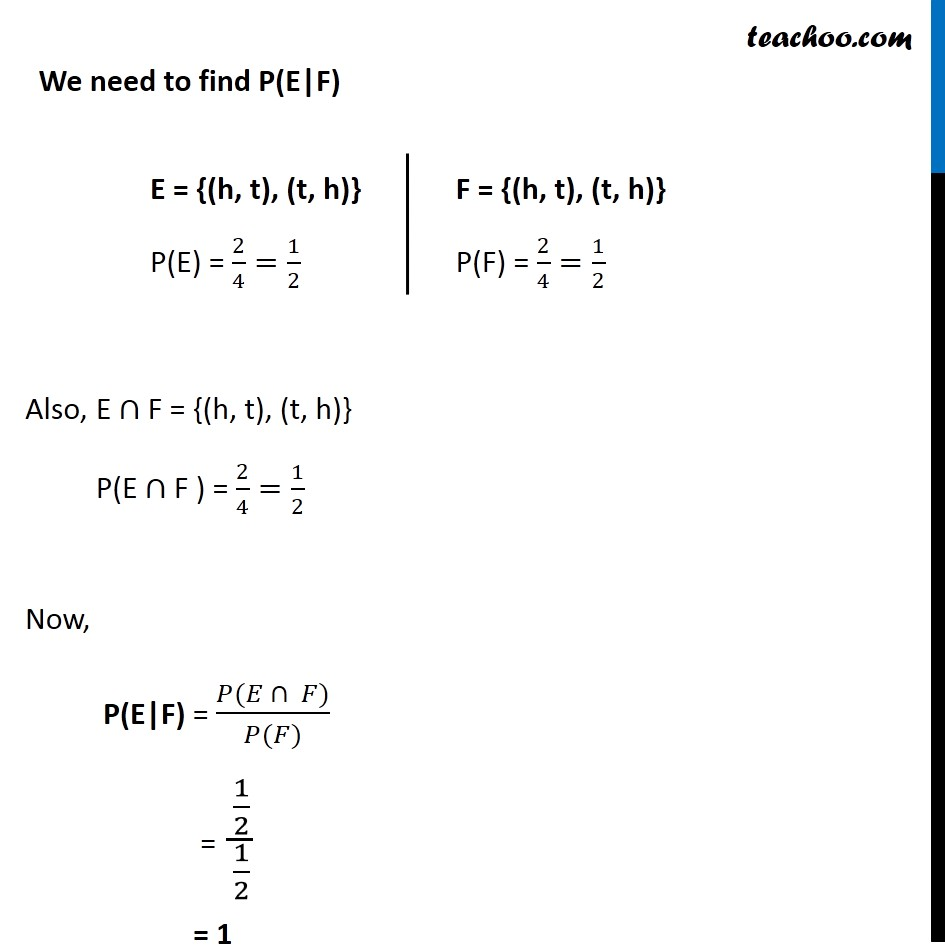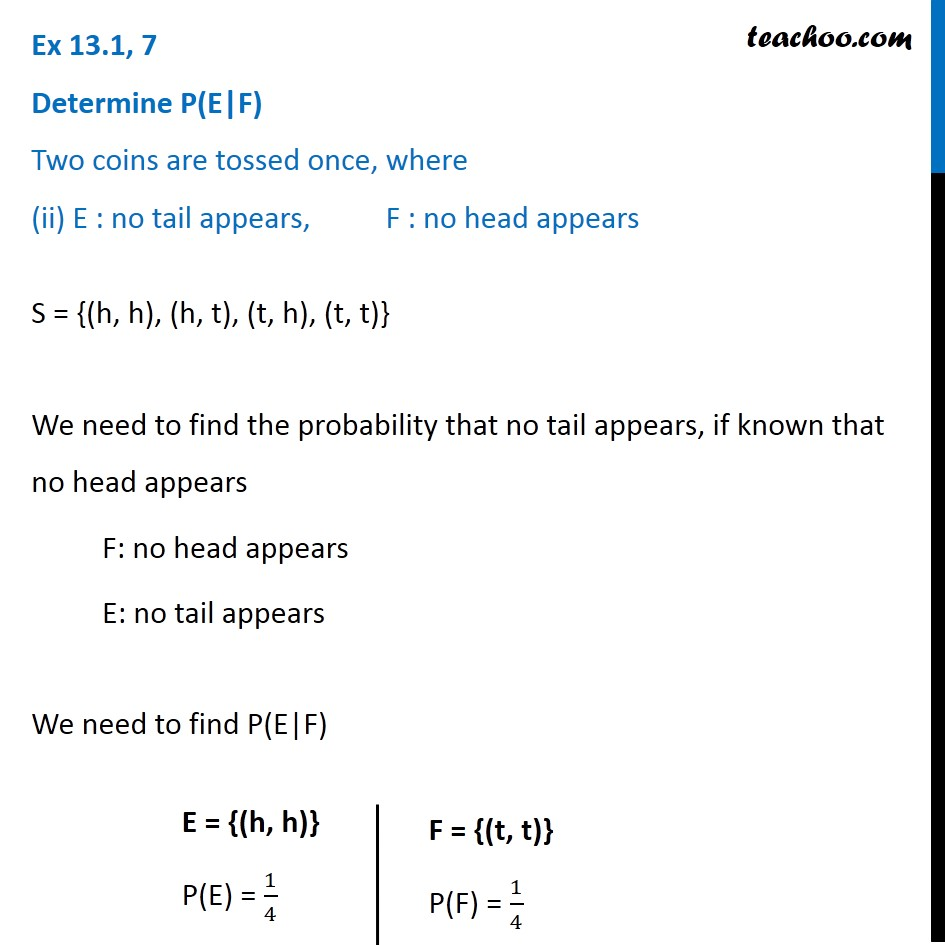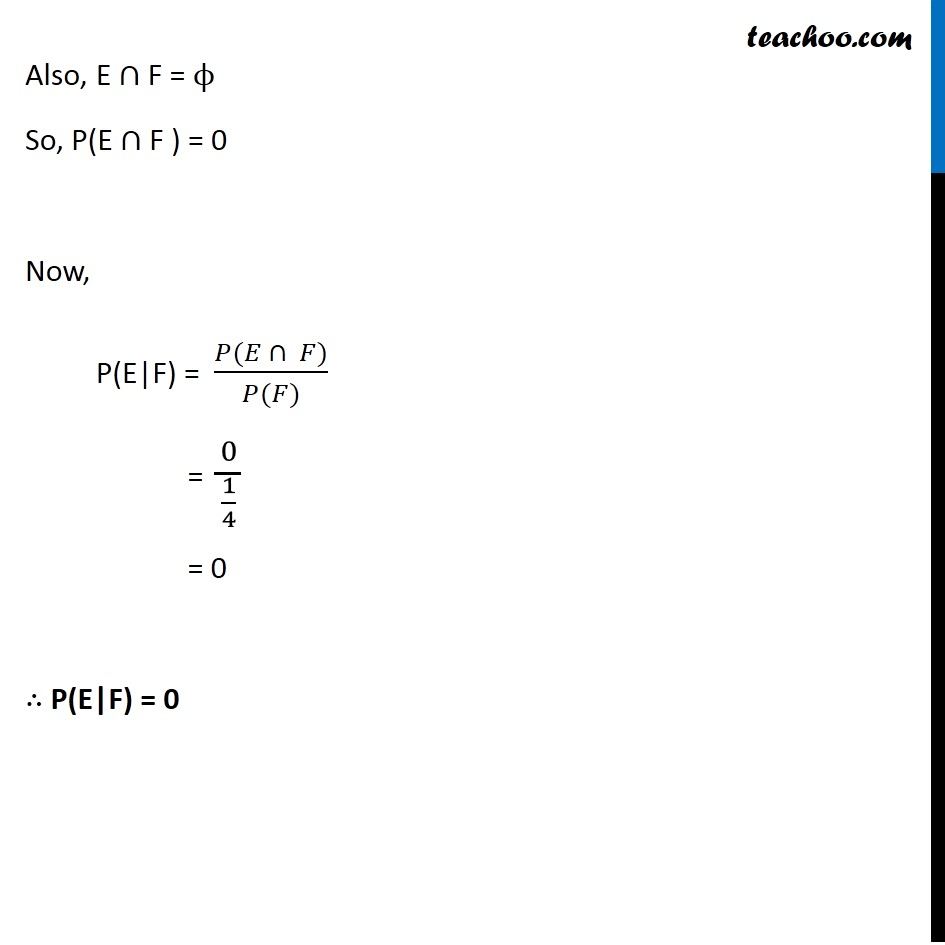Subscribe to our Youtube Channel - https://you.tube/teachoo

1. Chapter 13 Class 12 Probability
2. Serial order wise
3. Ex 13.1

Transcript

Ex 13.1, 7 ﻿Determine P(E|F) Two coins are tossed once, where (i) E : tail appears on one coin, F : one coin shows headTwo coins are tossed once Let heads be denoted by ‘h’ & tails be denoted by ‘t’ S = {(h, h), (h, t), (t, h), (t, t)} We need to find the probability that tail appears on one coin, if we know that one coin shows head Let F: one coin shows head E : tail appears on one coin E = {(h, t), (t, h)} P(E) = 2/4=1/2 E = {(h, t), (t, h)} P(E) = 2/4=1/2 F = {(h, t), (t, h)} P(F) = 2/4=1/2 Also, E ∩ F = {(h, t), (t, h)} P(E ∩ F ) = 2/4=1/2 Now, P(E|F) = (𝑃(𝐸 ∩ 𝐹))/(𝑃(𝐹)) = (1/2)/(1/2) = 1 Ex 13.1, 7 ﻿Determine P(E|F) Two coins are tossed once, where (ii) E : no tail appears, F : no head appears S = {(h, h), (h, t), (t, h), (t, t)} We need to find the probability that no tail appears, if known that no head appears F: no head appears E: no tail appears We need to find P(E|F) E = {(h, h)} P(E) = 1/4 F = {(t, t)} P(F) = 1/4 Also, E ∩ F = ϕ So, P(E ∩ F ) = 0 Now, P(E|F) = (𝑃(𝐸 ∩ 𝐹))/(𝑃(𝐹)) = 0/(1/4) = 0 ∴ P(E|F) = 0

Ex 13.1